A gear is a simple mechanical device that transmits rotational motion (force) to another gear or device. Gears are round and have teeth—linkages to mesh with other teeth—leading to a relatively slip-free transfer of movement. The amount of torque, speed, and direction of movement depends upon the gear’s design; function follows form in the transfer of forces in gears. When combined with other gears of different diameters, a mechanical advantage—and, thus, work can be performed—can be produced due to the gears’ differing torque and rotational speed. These different tooth/gear ratios give rise to different speeds in overall transfer of force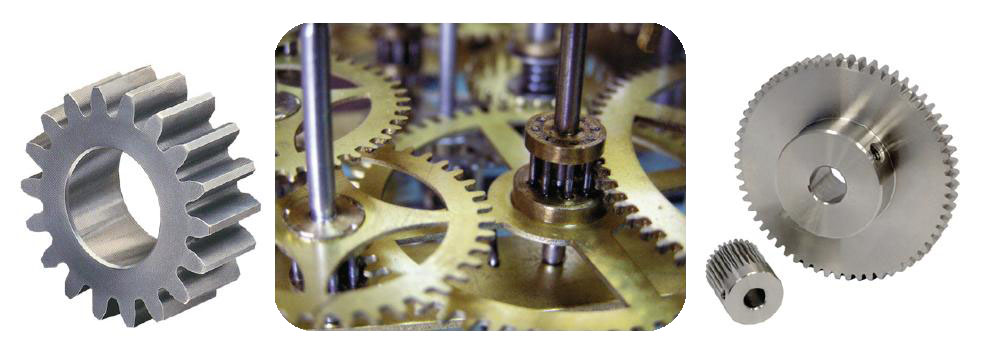Gears

 Thus, the larger a gear’s radius, the slower its rotational speed.

 Gear Ratios When the teeth of two gears interlock, the gears themselves move at the same speed (meters per second or feet per minute). Rotational speed is proportional to the circumferential speed of a wheel—circle—divided by its radius.

 Counting Teeth In a small/large gear set-up, when the small gear has made a full rotation, the large gear will not have yet completed a full rotation. Thus, the smaller gear makes more revolutions in a given time, rotating at a faster pace than the larger gear. For two given gears, one of number of teeth A and one of number of teeth B, the speed ratio (gear ratio) is as follows: (Speed A * Number of teeth A) = (Speed B * Number of teeth B)

 Forces in Gears When two gears are touching, the force of one gear’s teeth exerts force on the teeth of the other gear. Since the touching teeth produce a line joining the shaft axes of both the gears, this force will have both a radial and circumferential component. In calculations, the circumferential component is most important; the radial component merely causes a left-right push on the shaft, but does not contribute to the gears’ turning. Thus, the circumferential component of force gives rise to the gears’ rotational movement. The associated torque is quantified by multiplying the circumferential component by the radius; larger gears experience a greater amount of torque, whereas smaller gears experience less torque. Similarly, the torque ratio is equal to the ratio of the gears’ radii. Torque = (Force’s Circumferential Component) * (Gear Radius)

 Torque Ratio = (Radius A)/(Radius B) Comparing the aforementioned velocity ratio (see “Counting Teeth”) with the torque ratio illustrates that both ratios are inverses of each other. Thus, a higher velocity ratio implies a lower torque ratio, and a lower velocity ratio implies a higher torque ratio.

 Useful Gear Nomenclature Point of Contact Any point where two tooth profiles are touching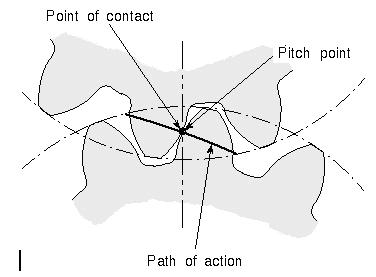Point of Contact

 Line of Contact A line or curve produced by two teeth tangent to each other over a distance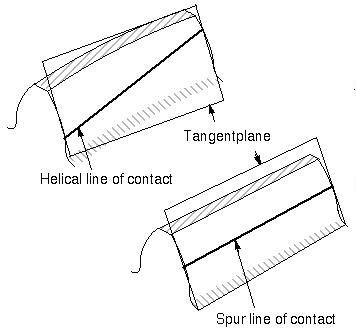Line of Contact

 Line of Action During movement, the succession of contact points between two teeth. In involute gears (the most common type of teeth profile), the path of action is the same as the line of action. Thus, the line of action is the straight line passing through the pitch point that is also tangent to both gears.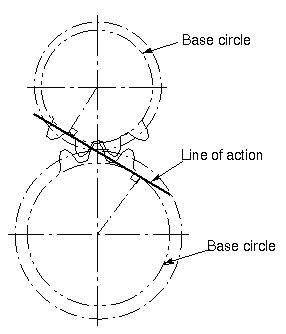Line of Action

 Pitch Point The point where the line of action crosses the line joining the two gear axes.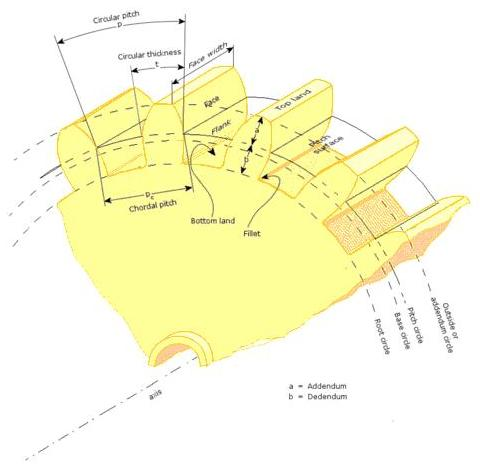Summary of Gear Parts

 Gears on Our EPICS Time Team Clock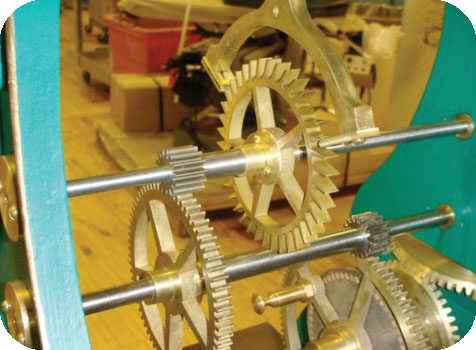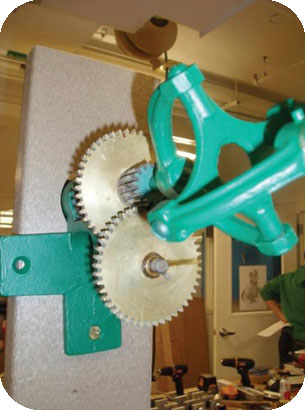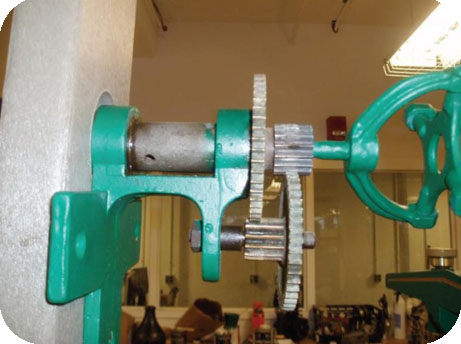Sources: American National Standards Institute (ANSI) / American Gear Manufacturers Association (AGMA) 1012-G05, "Gear Nomenclature, Definition of Terms with Symbols". McGraw Hill Encyclopedia of Science and Technology, “Gear”, 742-3. McGraw Hill: 2002. http://auto.howstuffworks.com/cvt1.htm http://en.wikipedia.org/wiki/Gear#Line_of_action http://ocw.mit.edu/OcwWeb/Electrical-Engineering-and-Computer-Science/6-883Fall-2005/CourseHome/ http://www.precisiongears.com/images/spur_gear.jpg http://www.rpmechatronics.co.uk/images/products/gear-range.jpg

Written by Stephanie Bachas-Daunert'10